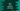# Java program to read and print a two dimensional array### Java program to read and print a two-dimensional array :

In this tutorial, we will learn how to read elements of a two-dimensional array and print out the result. We will first read the row and column number from the user and then we will read all elements one by one using a loop.

Let’s take a look at the algorithm first :

### Algorithm :

1. Read the row and column number first.

2. Create one two dimensional array to hold the numbers.

3. Using a for loop read all numbers and store it in the array.

4. After the reading is completed, print out the numbers using an array.

### Java program :

``````import java.util.Scanner;

public class Main {

public static void main(String[] args) {

//1
int row, col;

//2
int arr[][];

//3
Scanner scanner = new Scanner(System.in);

//4
System.out.print("Enter the total number of row : ");

//5
row = scanner.nextInt();

//6
System.out.print("Enter the total number of column : ");
col = scanner.nextInt();

//7
arr = new int[row][col];

//8
for (int i = 0; i < row; i++) {
//9
for (int j = 0; j < col; j++) {
//10
System.out.print("Enter element for row = " + (i + 1) + " column = " + (j + 1) + " : ");
arr[i][j] = scanner.nextInt();
}
}

//11
System.out.println("You have entered the following array : ");

//12
for (int i = 0; i < row; i++) {
for (int j = 0; j < col; j++) {
System.out.print(arr[i][j] + " ");
}
System.out.println();
}
}

}

``````

### Explanation :

The commented numbers in the above program denote the step number below :

1. Create two variables to store the row and column numbers: row and col.

2. Create one two dimensional array arr[][] . The first [] denotes the row count and the second [] denotes column count.

3. Create one scanner object to read the user input values.

4. Ask the user to enter the row count.

5. Put the row count in row variable.

6. Similarly, ask the user to enter the column value and store it in the col variable.

7. Create one two dimensional array with row count as row and column count as col and assign it to the variable arr.

8. Start one for loop to run for row times.

9. Inside that loop, run one more loop and run it for col times.

10. Ask the user to enter one number and store it in [i][j] position. After both loops are completed, the array arr will hold all elements that user has entered.

11. Now, print out the entered array to the user.

12. Similarly, run two for loops and print out all numbers of arr.

### Sample Output :

``````Enter the total number of row : 3
Enter the total number of column : 4
Enter element for row = 1 column = 1 : 1
Enter element for row = 1 column = 2 : 2
Enter element for row = 1 column = 3 : 3
Enter element for row = 1 column = 4 : 4
Enter element for row = 2 column = 1 : 5
Enter element for row = 2 column = 2 : 6
Enter element for row = 2 column = 3 : 7
Enter element for row = 2 column = 4 : 8
Enter element for row = 3 column = 1 : 9
Enter element for row = 3 column = 2 : 10
Enter element for row = 3 column = 3 : 11
Enter element for row = 3 column = 4 : 12
You have entered the following array :
1 2 3 4
5 6 7 8
9 10 11 12
``````